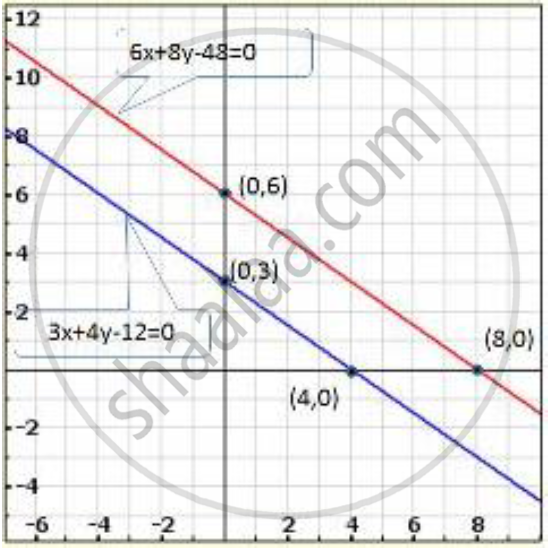Share

# The Path of a Train a is Given by the Equation 3x + 4y − 12 = 0 and the Path of Another Train B is Given by the Equation 6x + 8y − 48 = 0. Represent this Situation Graphically. - CBSE Class 10 - Mathematics

ConceptGraphical Method of Solution of a Pair of Linear Equations

#### Question

The path of a train A is given by the equation 3x + 4y − 12 = 0 and the path of another train B is given by the equation 6x + 8y − 48 = 0. Represent this situation graphically.

#### Solution

The paths of two trains are given by the following pair of linear equations.

3x + 4y - 12 = 0 ...(1)

6x + 8y - 48 = 0 ...(2)

In order to represent the above pair of linear equations graphically. We need two points on the line representing each equation. That is, we find two solutions of each equation as given below:

We have

3x + 4y - 12 = 0

Putting y = 0, we get

3x + 4 xx 0 - 12 = 0

=> 3x = 12

=> x = 12/3 = 4

Putting x = 0, we get

3 xx 0 + 4y - 12 = .0

=> 4y = 12

=> y = 12/4 = 3

Thus, two solution of equation 3x + 4y - 12 = 0 are (0.3) and (4,0)

We have

6x + 8y - 48 = 0

Putting x = 0 we get

6 xx 0 + 8y - 48 = 0

=> 8y = 48

=> y = 48/8

=> = 6

Putting y = 0 we get

6x + 8 xx 0  = 48 = 0

=> 6x = 48

=> x = 48/6 = 8`

Thus, two solution of equation 6x + 8y - 48 = 0 are (0, 6) and (8,0)Clearly, two lines intersect at (-1, 2)

Hence, x = -1, y = 2 is the solution of the given system of equations.

Is there an error in this question or solution?

#### APPEARS IN

Solution The Path of a Train a is Given by the Equation 3x + 4y − 12 = 0 and the Path of Another Train B is Given by the Equation 6x + 8y − 48 = 0. Represent this Situation Graphically. Concept: Graphical Method of Solution of a Pair of Linear Equations.
S